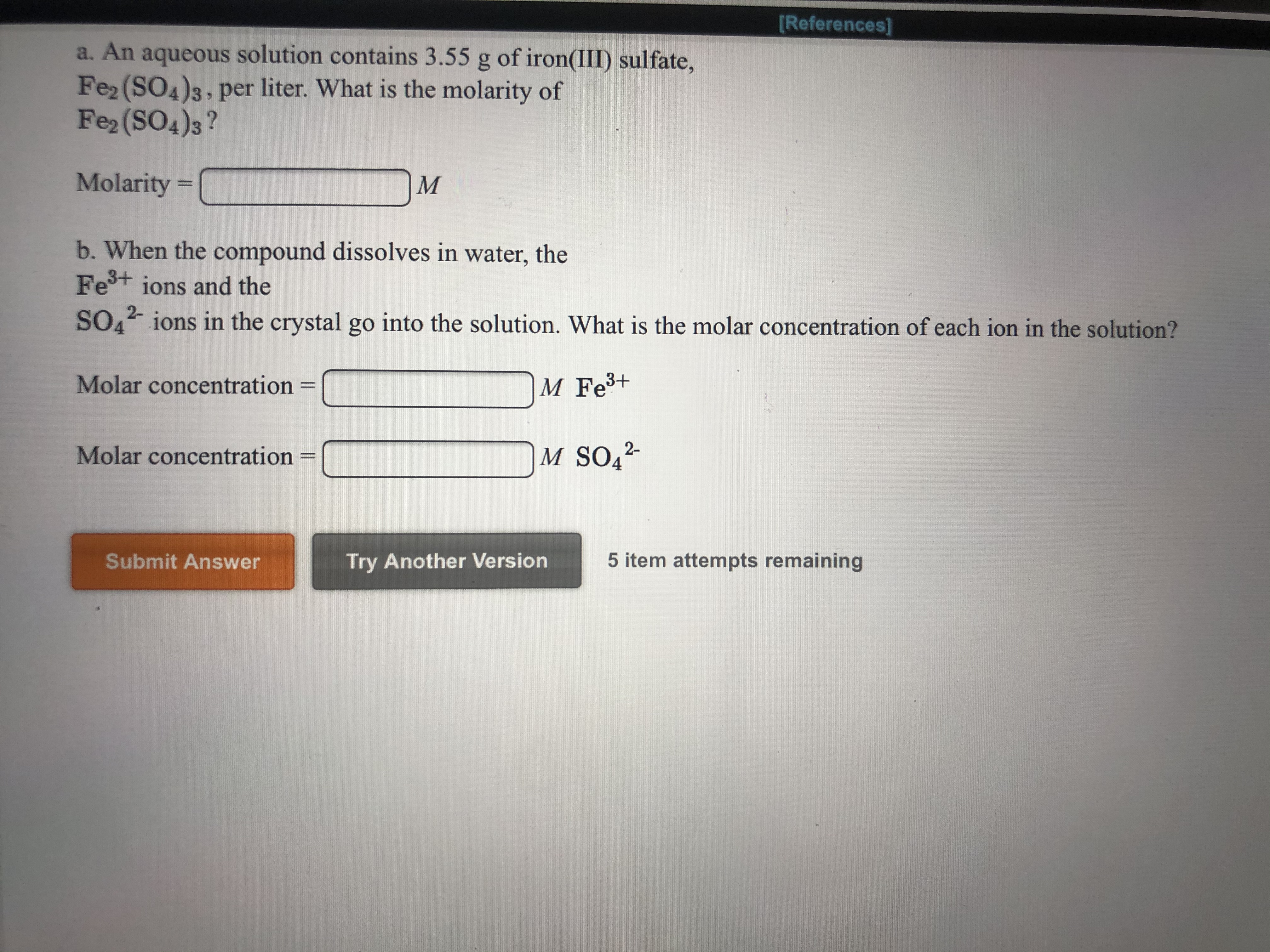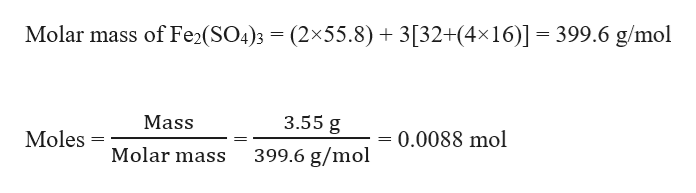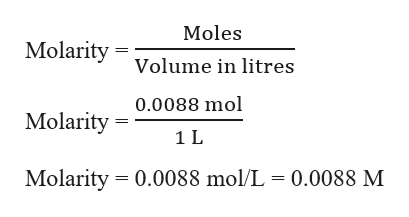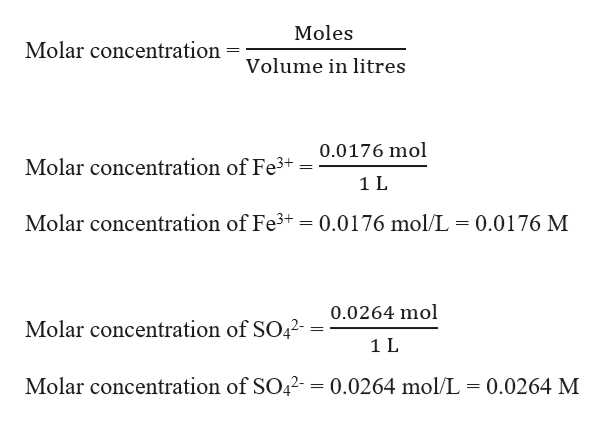# References]a. An aqueous solution contains 3.55 g of iron(III) sulfate,Fe2 (SO4)3, per liter. What is the molarity ofFe2 (SO4)3?Molarity =Мb. When the compound dissolves in water, theFe+ ions and the2-SOO ions in the crystal go into the solution. What is the molar concentration of each ion in the solution?Molar concentrationM Fe3+M SO42Molar concentration5 item attempts remainingSubmit AnswerTry Another Version

Question
3 viewshelp_outlineImage TranscriptioncloseReferences] a. An aqueous solution contains 3.55 g of iron(III) sulfate, Fe2 (SO4)3, per liter. What is the molarity of Fe2 (SO4)3? Molarity = М b. When the compound dissolves in water, the Fe+ ions and the 2- SOO ions in the crystal go into the solution. What is the molar concentration of each ion in the solution? Molar concentration M Fe3+ M SO42 Molar concentration 5 item attempts remaining Submit Answer Try Another Version fullscreen
check_circle

Step 1

(a). Given,

Mass of iron(III) sulfate, Fe2(SO4)3 = 3.55 g

Volume of solution = 1 L

Moles of iron(III) sulfate, Fe2(SO4)3 can be calculated as:help_outlineImage TranscriptioncloseMolar mass of Fe2(SO4)3 (2x55.8) 3[32+(4x16)] 399.6 g/mol 3.55 g Mass Moles = 0.0088 mol Molar mass 399.6 g/mol fullscreen
Step 2

Molarity of Fe2(SO4)3 solution can be calculated as:help_outlineImage TranscriptioncloseMoles Molarity Volume in litres 0.0088 mol Molarity 1 L Molarity 0.0088 mol/L = 0.0088 M fullscreen
Step 3

(b). Given, when Fe2(SO4)3 dissolves in water Fe3+ ions and SO42- ions in the crystal go into the solution.

This can be written as a balanced reaction as :

Fe2(SO4)3 → 2 Fe3+ + 3 SO42-

1 mole of Fe2(SO4)3 gives 2 moles of Fe3+ and 3 moles of SO42-

Therefore, 0.0088 mol of Fe2(SO4)3 will give:

2 × 0.0088 moles = 0.0176 moles of Fe3+ and

...help_outlineImage TranscriptioncloseMoles Molar concentration Volume in litres 0.0176 mol Molar concentration of Fe3 1L Molar concentration of Fe3+ 0.0176 mol/L 0.0176 M 0.0264 mol Molar concentration of SO42-- 1 L Molar concentration of SO42- = 0.0264 mol/L = 0.0264 M fullscreen

### Want to see the full answer?

See Solution

#### Want to see this answer and more?

Solutions are written by subject experts who are available 24/7. Questions are typically answered within 1 hour.*

See Solution
*Response times may vary by subject and question.
Tagged in

### Solutions